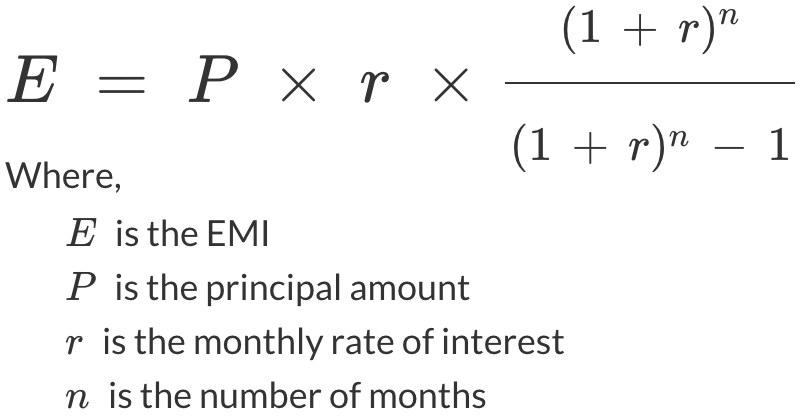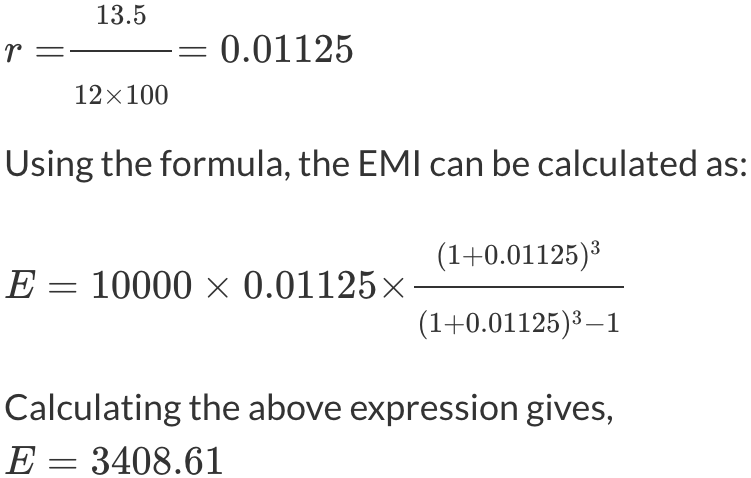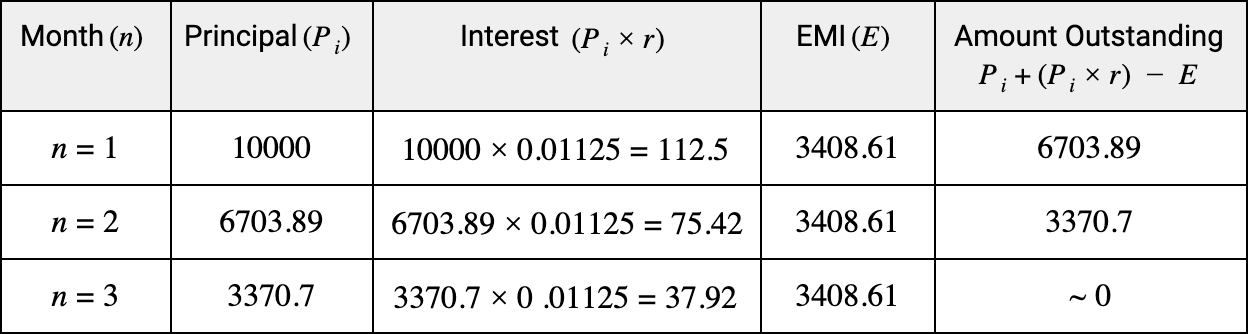# Instant Loan EMI Calculator

• 0
• 20k
• 40k
• 60k
• 80k
• 100k
• 120k
• 140k
• 160k
• 180k
• 200k
• 0%
• 5%
• 10%
• 15%
• 20%
• 25%
• 30%
• 35%
• 0
• 1
• 2
• 3
• 4
• 5
• 6
• 7
• 8
• 9
• 10
• 11
• 12
EMI:  ₹0
Total Interest:  ₹0

### What is EMI?

EMI or Equated Monthly Installment is the fixed amount paid by a borrower on a monthly basis to the lender. It is generally paid by the customer on a particular calendar date until the principal amount and the total interest accrued on it for the loan duration are paid off. The EMI amount is calculated in such a way that the interest of the loan is larger during the initial months and reduces gradually with each payment. Key parameters used in EMI calculation for an instant loan are:

• Principal amount: The base amount that is lent to the customer as loan is called the principal amount. It may differ from lender to lender based on their evaluation of the customer’s credit profile. The principal amount for an instant loan is typically upto ₹200000.
• Interest Rate: The rate of interest is the percentage which can be levied on the principal amount of a loan on a periodic basis as a proportion of the amount lent which can be upto 35% for instant loans.
• Loan Duration: The loan duration or loan term, also commonly referred to as “tenor” is the period for which a loan is sanctioned. The borrower must pay the principal sum and the total interest within the loan duration. The tenor for instant loan is typically between 3 - 12 months.

### What is an instant loan?

Instant loan is a quick, short-term loan of small amount which can be taken for any personal reason. The loan processing is much faster and the money gets disbursed quickly.

### How is the EMI calculated for instant loan?

EMI for instant loan can be calculated as:You can check the derivation of EMI calculation formula to learn more.

### Example of EMI calculation

Assume you get an instant loan of ₹10,000 at an annual interest rate of 13.5% for a period of 3 months.
The monthly interest rate becomes:which means you need to ₹3,408.61 as EMI for a loan of ₹10,000 for 3 months if borrowed at 13.5% interest rate.

Now, let’s look at the EMI and interest breakdown with each installment:As we can see, the amount outstanding at the end of 3 months becomes zero which means the entire loan amount along with interest gets paid. We also observe that the interest component reduces after every month.
The total interest paid during the loan tenor is 112.5 + 75.42 + 37.92 = 225.84

### How to use Credy’s Instant Loan EMI Calculator?

1. Use the first slider to enter the instant loan amount or enter in the input box directly.
2. Use the second slider to enter the annual rate of interest or enter in the input box directly.
3. Use the third slider to enter the loan duration in months or enter in the input box directly.
4. The value of calculated EMI, total interest, total payable amount and breakdown of the total payable amount will be displayed if all three values were entered correctly.

### Benefits of using Instant Loan EMI Calculator

1. Get a loan within your budget:
EMI is the fixed monthly amount that needs to be paid in order to borrow a certain amount of loan. Hence, the EMI calculator helps plan the instant loan amount based on your current obligations, expenses, and monthly budget by calculating the amount that can be paid comfortably on a regular basis.

2. Decide the loan tenor:
If the calculated EMI exceeds your monthly capacity, then you could review your instant loan tenor and choose a more suitable one. Instant loans, however, are unsecured loans which may come with high interest rates. It is therefore advisable to opt for the lowest tenor of the loan so as to have minimal interest.

3. Calculate your eligibility for a loan:
Once you have your loan requirements sorted and EMI calculated, you can check if you are eligible to get that loan or not. Credy has a very simple 2-step eligibility check process that requires you to fill only basic details and quickly evaluates your eligibility for the instant loan you are looking for.